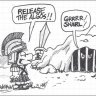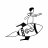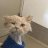# Scanner predicting a stocks movement for the next few days?##### Well-known member
I've been successful in making trades but it takes me ages to find the stocks with the best set-ups. Any savvy coders or people who know the code to a scanner(s) that would help me with this?. I usually swing trade in the 1 to 3 week range but really just when I have high confidence to when I have low confidence in the chart. Thank You.

D

#### drdarshil16

##### New member
2019 Donor
@YungTraderFromMontana YOU CAN PLACE YOUR CRITERIA ON HOW YOU TRADE AND SOMEONE CAN COME UP WITH SCANNER

•BenTen#### horserider

##### Well-known member
VIP
I wish I did !!!!!!•RDMercer and drdarshil16#### BenTen

Staff
VIP
I agree with @drdarshil16. Questions like this don't usually get a lot of feedback from the community because they're so general. Since you're a swing trader and you need a way to figure out when a stock will make a big move, maybe this can help?

If you have a specific condition on how to find such stocks, I would suggest writing it here so we can help you come up with a scanner for it.

•markos##### Well-known member
Okay thank you, I added blast off to my arsenal. Is there anyway you could combine the Ultimate Macd by Horserider, True Momentum oscillator by Mobius, and Advanced Market Forecast Indicator by Dilbert/Stanl/Mobiusto to trigger a signal when 1. the Macd Line is green, 2. the oscillator line shows green at least 5 below the zero(-5 y axis) and 3. the advanced market forecast indicator shows intermediate line below 25 on the y axis, then I'm going to test that and see if I can find solutions to failed signals. Do you want me to paste the code here or can you find them? They both have high views on here.
Now that I think about it can you make it into a arrowed signal that can be converted into a scanner. My coding ability isn't that good or I would've tackled this. I really appreciate any help.

Edit: I may eventually need to figure out a way to eliminate bias based on the trend and then I could shrink the range of the criteria and make it even more applicable. Also next on the list would be a sell signal.

Last edited:##### Member
I've been successful in making trades but it takes me ages to find the stocks with the best set-ups. Any savvy coders or people who know the code to a scanner(s) that would help me with this?. I usually swing trade in the 1 to 3 week range but really just when I have high confidence to when I have low confidence in the chart. Thank You.

RSI LAguerre +Hull Works well for my style

https://tos.mx/pfhCWOC
Fundamentlas Russell3000 + TMO+Coppock+some filters
https://tos.mx/Q7q54ME

•jacqshen
S

#### skynetgen

##### Well-known member
"Do you have a formula to print money?" Did I get the question right?##### Member
"Do you have a formula to print money?" Did I get the question right?
Sorry..i don´t undersatand your comment, i only try to help with this scans works well for me##### Well-known member
@Ugautrader much appreciated will check it out.#### markos

##### Well-known member
VIP
@Ugautrader much appreciated will check it out.
@YungTraderFromMontana Those three criteria could be done by yourself by using the scanning wizard.
Please look in the Tutorial section for How To Drive the Scanner. The videos there should get you started.
It is alot of code for someone to just pull up to create a scan for you to then test it.
Why did you pick those three studies?
What type of stocks do you look for? Or is it options or futures?
Let us know how you are progressing on your quest.##### Well-known member
Thanks for the response, I found a way to get code working with the criteria. I look for stocks that fit my criteria and have low option spreads, so options. Here is a sloppy conglomeration of indicators and one line of plot at the bottom that completes the scan. Definitely not the best way to do this but gets the job done.
Rich (BB code):
``````declare lower;
input ImedLength = 31; # orig std = 31
input MomentumLength = 5; # orig std = 31
input NearLength = 3; # orig std = 3
input length = 14;
input calcLength = 5;
input smoothLength = 3;

input fastLength = 6;
input slowLength = 13;
input MACDLength = 6;
input AverageTypeMACD = {SMA, default EMA, Wilders};

input price = close;
input displace = 0;

def MACD_Data = MACD(fastLength = fastLength, slowLength = slowLength, MACDLength = MACDLength);
def MACD_Line = MACD_Data;

def Value;
def Avg;
switch (AverageTypeMACD) {
case SMA:
Value = Average(price, fastLength) - Average(price, slowLength);
Avg = Average(Value, MACDLength);
case EMA:
Value = ExpAverage(price, fastLength) - ExpAverage(price, slowLength);
Avg = ExpAverage(Value, MACDLength);
case Wilders:
Value = WildersAverage(price, fastLength) - WildersAverage(price, slowLength);
Avg = ExpAverage(Value, MACDLength);
}

#plot BB;
#Bollinger BandsSMA,EMA
input AverageTypeBB = {default SMA, EMA, HMA};
input displaceBB = 0;
input lengthBB = 20;
input Num_Dev_Dn = -2.0;
input Num_Dev_up = 2.0;

def upperBand;
def lowerBand;
def midline;

switch (AverageTypeBB) {
case SMA:
upperBand = reference BollingerBands(MACD_Line, displaceBB, lengthBB, Num_Dev_Dn, Num_Dev_up).UpperBand;
lowerBand = reference BollingerBands(MACD_Line, displaceBB, lengthBB, Num_Dev_Dn, Num_Dev_up).LowerBand;
midline   = reference BollingerBands(MACD_Line, displaceBB, lengthBB, Num_Dev_Dn, Num_Dev_up).Midline;
case EMA:
upperBand = reference BollingerBands(MACD_Line, displaceBB, lengthBB, Num_Dev_Dn, Num_Dev_up, averageType = AverageType.EXPONENTIAL).UpperBand;
lowerBand = reference BollingerBands(MACD_Line, displaceBB, lengthBB, Num_Dev_Dn, Num_Dev_up, averageType = AverageType.EXPONENTIAL).LowerBand;
midline   = reference BollingerBands(MACD_Line, displaceBB, lengthBB, Num_Dev_Dn, Num_Dev_up, averageType = AverageType.EXPONENTIAL).Midline;
case HMA:
upperBand = reference BollingerBands(MACD_Line, displaceBB, lengthBB, Num_Dev_Dn, Num_Dev_up, averageType = AverageType.EXPONENTIAL).UpperBand;
lowerBand = reference BollingerBands(MACD_Line, displaceBB, lengthBB, Num_Dev_Dn, Num_Dev_up, averageType = AverageType.EXPONENTIAL).LowerBand;
midline   = reference BollingerBands(MACD_Line, displaceBB, lengthBB, Num_Dev_Dn, Num_Dev_up, averageType = AverageType.EXPONENTIAL).Midline;
}

def midline1 = midline;

def o = open;
def c = close;
def dataa = fold i = 0 to length
with s
do s + (if c > GetValue(o, i)
then 1
else if c < GetValue(o, i)
then - 1
else 0);
def EMA5 = ExpAverage(dataa, calcLength);
def Main = ExpAverage(EMA5, smoothLength);
def Signal = ExpAverage(Main, smoothLength);

def zero = if IsNaN(c) then Double.NaN else 0;

def ob = if IsNaN(c) then Double.NaN else Round(length * .7);

def os = if IsNaN(c) then Double.NaN else -Round(length * .7);

def c1I = close - Lowest(low, ImedLength);
def c2I = Highest(high, ImedLength) - Lowest(low, ImedLength);
def FastK_I = c1I / c2I * 100;
def c1N = close - Lowest(low, NearLength);
def c2N = Highest(high, NearLength) - Lowest(low, NearLength);
def FastK_N = c1N / c2N * 100;

def c1M = close - Lowest(low, MomentumLength);
def c2M = Highest(high, MomentumLength) - Lowest(low, MomentumLength);
def FastK_M = c1M / c2M * 100;
def Intermediate = Average(FastK_I, 5); #  = TMF's GreenLine

def NearTerm = Average(FastK_N, 2);     #  = TMF's BlueLine

def Min1 = Min(low, low);
def Min2 = Min(Min1, Min(low, low));
def Max1 = Max(high, Max(high, Max(high, high)));

def momentum = ((close - Min1) / (Max1 - Min2)) * 100;

def bottomline = 0;

def topLine = 100;

def UpperRevZone = 80;

def LowerRevZone = 40;

def UpLimit = 120;

plot data = (Intermediate < LowerRevZone) and (Main > Signal) and (-length <= ob + 5) and (upperBand > upperBand and lowerBand < lowerBand);``````

Last edited:
•markos and BenTenIs it possible to create a scanner for new daily highs? Questions 0On Balance Volume Scanner Questions 12